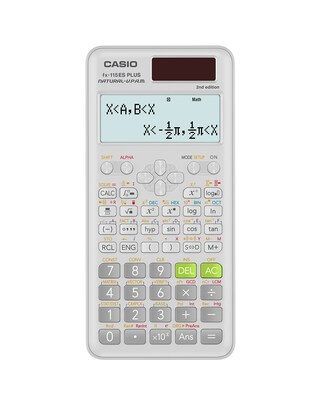Shop Products
Shop by Brand

Quill+

Members get the lowest \$34.99 paper price + free shipping.

CloseFree Shipping. No Minimum.

Code: FREESHIP

Offer good through 10/2/22.

# Casio 2nd Edition 16-Digit Solar Powered Scientific Calculator, White (FX-115ESPLS2-S)

Item #: 901-24431368
Model #: FX-115ESPLS2-S
Brand: Casio\$20.99 Each
Enter Zip Code

Estimated Delivery Date:
Customers Also Viewed
Description
Casio 2nd Edition FX-115ESPLS2-S is an advanced solar-plus-battery scientific calculator that provides long life and dependability. With Natural Textbook Display, in addition to advance statistics, matrix, and conversion functionality, it's a perfect choice for high school and college students. Recommended for students taking general math, pre-algebra, algebra I and II, geometry, trigonometry, statistics, calculus, chemistry, and engineering.
• Scientific calculator with two-line Natural Textbook Display displays formulas and results simultaneously
• Perfect choice for high school and college students taking general math, pre-algebra, algebra I and II, geometry, trigonometry, statistics, calculus, chemistry, engineering
• Dimensions: 0.44"H x 3.15"W x 6.38"D
• Calculator is solar cell-powered
• 417 built-in functions
• Performs the following: addition, subtraction, multiplication, division, augmentation, absolute value, reciprocal, square, cube, and conjugate of complex number calculations
• Features linear regression, quadratic regression, logarithmic regression, e-exponential regression, ab-exponential regression, power regression, and inverse regression
• Can transform a displayed decimal calculation result to a fraction or mixed number
• Fraction/decimal conversions, simplifying fractions and roots, recurring decimal display, remainder calculation, integer division with remainder
• Prime factorization, metric conversion, hyperbolic functions, greatest common divisor and least common multiple, random integer generator, summation notation, verify computations
• Solves simultaneous linear equations with two unknowns, simultaneous linear equations with three unknowns, quadratic equations, and cubic equations
• Matrix mode allows you to perform matrix operations on matrices with up to three rows and three columns, also allowing you to enter vectors and perform vector calculations
• Features a new sleek design and hard slide-on case
• 1-year manufacturer limited warranty
Specifications
 Brand : Casio Color Family : White Customizable : No Depth in Inches : 6.38 Features : Sleek design, 2-line Natural Textbook Display, slide-on hard case Height in Inches : 0.44 Number of Digits Displayed : 16 Number of Lines : 4 Powered : Battery/Solar Powered Rechargeable : Rechargeable Series or Collection : 2nd Edition True Color : White Used For : General math, pre-algebra, algebra I and II, geometry, trigonometry, statistics, calculus, chemistry, engineering Warranty Information : 1 Year Weight (lbs.) : 0.23 Width in Inches : 3.15 Weight : 0.41 lbs. per EachLoading...